Checkout JEE MAINS 2022 Question Paper Analysis : Checkout JEE MAINS 2022 Question Paper Analysis :

# Triangles Class 10 Notes Chapter 6

## Class 10 Maths Chapter 6 Triangle Notes

The triangles class 10 notes chapter 6 provided here, is one of the most crucial study resources for the students studying in class 10. These CBSE chapter 6 notes are concise and cover all the concepts from this chapter from which questions might be included in the board exam. You will also come across theorems based on similar concepts. In your previous year classes, you must have learned about the basics of triangles such as the area of a triangle and its perimeters, etc.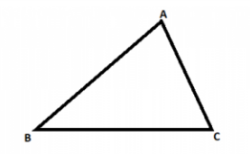The main concepts from this chapter that are covered here are-

• What is a triangle?
• Similarity criteria of two polygons having the same number of sides
• Similarity criteria of triangles
• Proof of Pythagoras Theorem
• Example Questions
• Problems based on Triangles
• Articles Related to Triangles

Students can refer to the short notes and MCQ questions along with separate solution pdf of this chapter for quick revision from the links below:

• Triangles Short Notes
• Triangles MCQ Practice Questions
• Triangles MCQ Practice Solutions
• ## What is Triangle?

A triangle can be defined as a polygon which has three angles and three sides. The interior angles of a triangle sum up to 180 degrees and the exterior angles sum up to 360 degrees. Depending upon the angle and its length, a triangle can be categorized in the following types-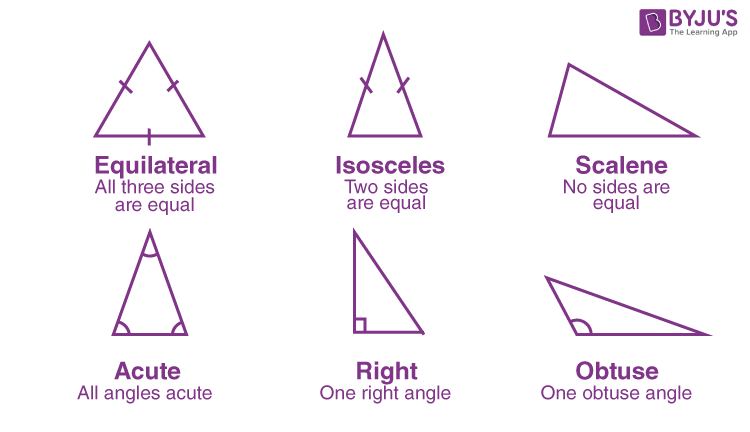1. Scalene Triangle – All the three sides of the triangle are of different measure
2. Isosceles Triangle – Any two sides of the triangle are of equal length
3. Equilateral Triangle – All the three sides of a triangle are equal and each angle measures 60 degrees
4. Acute angled Triangle – All the angles are smaller than 90 degrees
5. Right angle Triangle – Anyone of the three angles is equal to 90 degrees
6. Obtuse-angled Triangle – One of the angles is greater than 90 degreesTo know more about Triangles, visit here.

### Similarity Criteria of Two Polygons Having the Same Number of Sides

Any two polygons which have the same number of sides are similar if the following two criteria are met-

1. Their corresponding angles are equal, and
2. Their corresponding sides are in the same ratio (or proportion)

## Similarity Criteria of Triangles

Mathematical Expression for Similarity
In Δ ABC and Δ DEF, if
(i) ∠ A = ∠ D, ∠ B = ∠ E, ∠ C = ∠ F and
(ii)AB/DE = BC/ EF = CA/ FD , then the two triangles are similar.
To find whether the given two triangles are similar or not, it has four criteria. They are:

• Side-Side- Side (SSS) Similarity Criterion – When the corresponding sides of any two triangles are in the same ratio, then their corresponding angles will be equal and the triangle will be considered as similar triangles.
•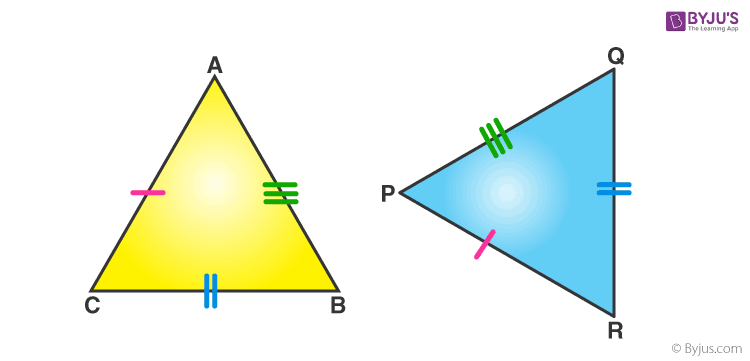• Angle Angle Angle (AAA) Similarity Criterion – When the corresponding angles of any two triangles are equal, then their corresponding side will be in the same ratio and the triangles are considered to be similar.
• Angle-Angle (AA) Similarity Criterion – When two angles of one triangle are respectively equal to the two angles of the other triangle, then the two triangles are considered as similar.
• Side-Angle-Side (SAS) Similarity Criterion – When one angle of a triangle is equal to one angle of another triangle and the sides including these angles are in the same ratio (proportional), then the triangles are said to be similar.
•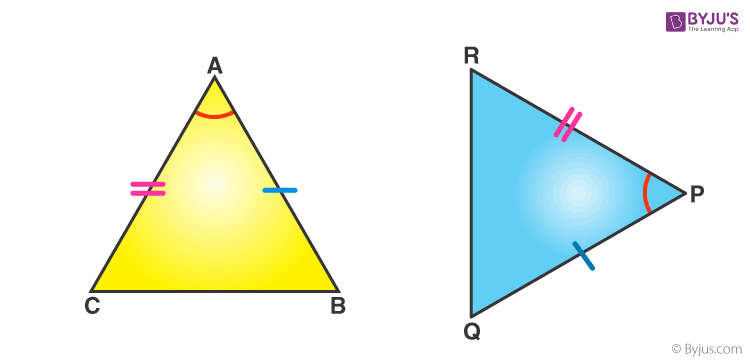To know more about Similar Triangles, visit here.

## Basic Proportionality Theorem

In Mathematics, the basic proportionality theorem states that “If a line is drawn parallel to one side of a triangle intersecting the other two sides in distinct points, then the other two sides are divided in the same ratio.”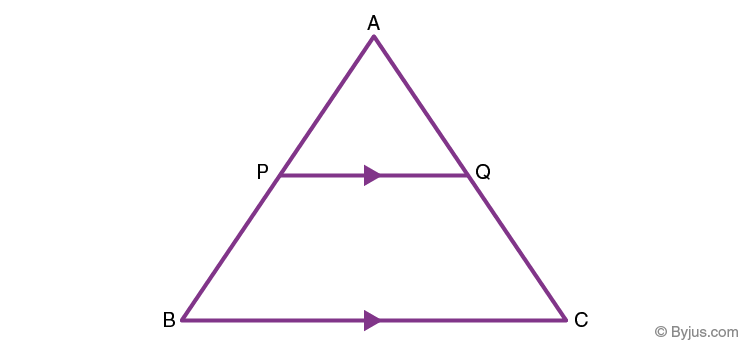Now, let us understand the Basic proportionality theorem with the help of a diagram.
Consider the triangle ABC, as depicted in the diagram. We draw a line PQ parallel to the side BC of ABC and intersecting the sides AB and AC in P and Q, respectively.
Thus, according to the Basic proportionality theorem,
AP/PB = AQ/QC

## Areas of Similar Triangles

According to the area of similar triangles theorem, “If two triangles are similar, then the ratio of the area of both triangles is proportional to the square of the ratio of their corresponding sides.”

Now, consider two triangles ΔABC and ΔPQR;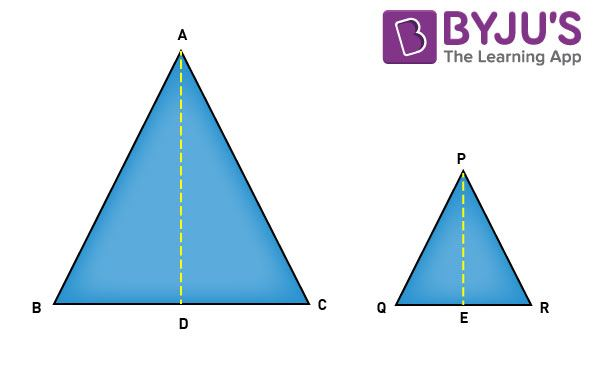Thus, according to the area of similar triangles, we can write:

Area of triangle ABC/Area of Triangle PQR = (AB/PQ)2 = (BC/QR) = (CA/RP)2

### Proof of Pythagoras Theorem

Statement: As per Pythagoras theorem“In a right-angled triangle, the sum of squares of two sides of a right triangle is equal to the square of the hypotenuse of the triangle.”

Pythagoras Theorem Formula

According to the Pythagoras theorem theorem, the formula is stated as follows:

Hypotneuse2 = Base2 + Perpendicular2

Now, consider the right triangle given below.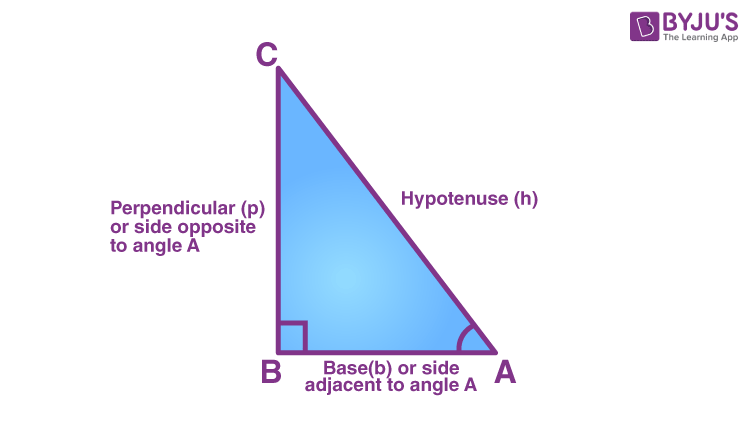Here, the side AC is the hypotenuse, which is the opposite to the right angle. It is longest side of a right triangle.

The side AB is the base, which is the side adjacent to angle A.

The BC is the perpendicular, which is the side opposite to angle A.

Proof –

Consider the right triangle, right-angled at B.

Construction-

Draw BD ⊥ AC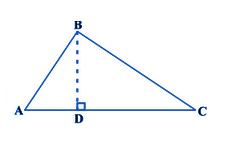or AD. AC = AB2 ……………(i)

Also, △BDC ~ △ ABC

So, CD/BC = BC/AC

or, CD. AC = BC2 ……………(ii)

AD. AC + CD. AC = AB2 + BC2

AC(AD + DC) = AB2 + BC2

AC(AC) = AB2 + BC2

⇒ AC2 = AB2 + BC2

Hence, proved.To know more about Pythagoras Theorem, visit here.

### Solved Example

Example 1:

In a right-angled triangle ABC, which is right-angled at A, where CM and BL are the medians of a triangle. Show that, 4(BL2 +CM2) = 5 BC2

Solution:

Given that,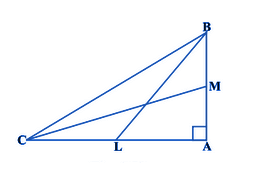Medians BL and CM,  ∠A = 90°

From the triangle ABC, we can write it as:

BC2 = AB2 + AC2 (Using Pythagoras Theorem) …(1)

From the triangle, ABL,

BL2 = AL2 +AB2

or we can write the above equation as:

BL2 =(AC/2)2 +AB2 (Where L is the midpoint of AC)

BL2 = (AC2/4) + AB2

4BL2 = AC2 + 4 AB2 ….(2)

From triangle CMA,

CM2 = AC2 + AM2

CM2 = AC2 + (AB/2)2 (Where M is the midpoint of AB)

CM2 = AC2 + AB2/4

4CM = 4 AC +AB ….(3)

Now, by adding (2) and (3), we  get,

4(BL2+ CM2) = 5(AC2+ AB2)

Using equation (1), we can write it as:

4(BL2+ CM2) = 5 BC2

Hence, it is proved.

Example 2:

In the given figure, DE is parallel to AC and DF is parallel to AE. Prove that BF/FE = BE/EC.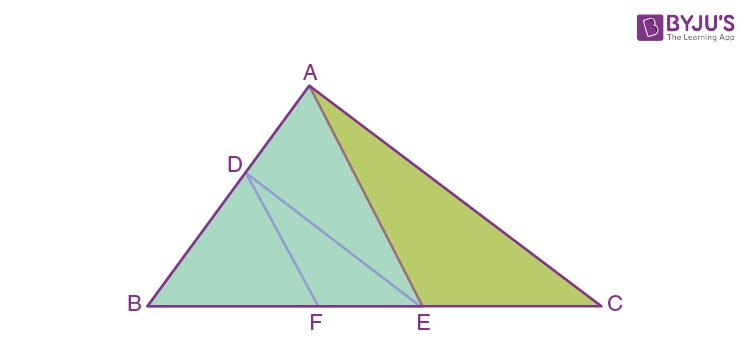Solution:

Given that DE || AC and DF||AE.

To prove: BF/FE = BE/EC

In a triangle ABC, DE || AC.

We know that the line drawn parallel to one side of a triangle, intersects the other two sides in distinct points, then it divides the other two sides in the same ratio.

Therefore, BE/EC = BD/DA …(1)

Now, consider the triangle AEB,

DF || AE.

Thus, we can say that

BF/FE = BD/DA …(2)

By comparing the equation (1) and (2), we can say

BE/EC = BF/FE

Hence, proved.

Example 3:

The sides AB and BC and median AD of a triangle ABC are respectively proportional to the sides PQ and QR and the median PM of a triangle PQR (as shown in the figure). Prove that △ABC ~ △PQR.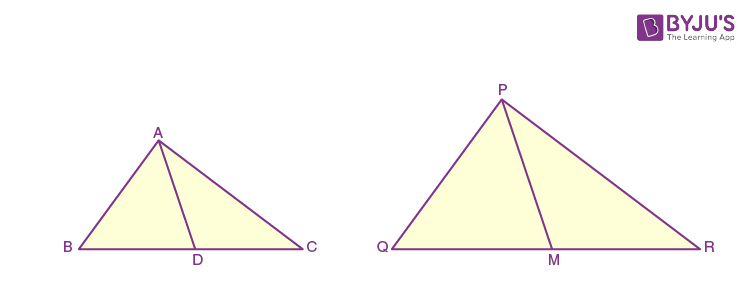Solution:

Given that,

In △PQR, PM is median

Also, it is provided that AB/PQ = BC/QR = AD/PM

Since, AD is the median of △ABC,

BD = DC = (½)BC

Similarly, PM is the median of △PQR,

QM = MR = (½)QR

Since, AB/PQ = BC/QR = AD/PM

AB/PQ = BD/QM = AD/PM ….(1)

Since, all the three sides are proportional, by using the SSS similarity rule, we can write

△ABD ~ △PQM

Since, the corresponding angles of similar triangles are equal

∠B = ∠Q …(2)

Therefore, in △ABC and △PQR,

∠B = ∠Q [From (2)]

AB/PQ = BC/QR [Given]

Therefore, by using SAS similarity, we can conclude that

△ABC ~ △PQR.

Hence, proved.

### Problems Related to Triangles

1. A girl having a height of 90 cm is walking away from a lamp-post’s base at a speed of 1.2 m/s. Calculate the length of that girl’s shadow after 4 seconds if the lamp is 3.6 m above the ground.
2. S and T are points on sides PR and QR of triangle PQR such that angle P = angle RTS. Now, prove that triangle RPQ and triangle RTS are similar.
3. E is a point on the side AD produced of a parallelogram ABCD and BE intersects CD
at F. Show that triangles ABE and CFB are similar.

Get more such class 10 maths and science notes at BYJU’S. Also, access class 10 question papers, sample papers, and other study materials to prepare for the board exam in a more effective way.

Quiz on Triangles Class 10

#### 1 Comment

1. Can u please provide me more questions of triangles chapters class 10th